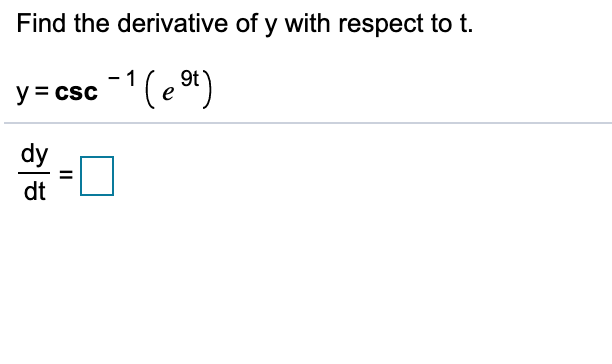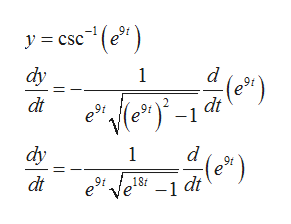# Find the derivative of y with respect to t-1y= csc(e 9t)dydtII

Question

Can you help me step by step?help_outlineImage TranscriptioncloseFind the derivative of y with respect to t -1 y= csc(e 9t) dy dt II fullscreen
check_circleExpert Solution
Step 1

We use chain rule of differentiation which states that if f(x)=g(h(x)), then f'(x)=g'(h(x))h'(x)

Step 2

By using the result that d/dx(csc^-1x)=-1/xsqrt(x^2-1)help_outlineImage Transcriptionclosey= csc(e") dy 1 d (e dt dt dy 1 (e*) dt 18t -1 dt fullscreen
Step 3

Now, we use the result t...

### Want to see the full answer?

See Solution

#### Want to see this answer and more?

Solutions are written by subject experts who are available 24/7. Questions are typically answered within 1 hour*

See Solution
*Response times may vary by subject and question
Tagged in

### Calculus